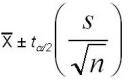## Fun with Confidence Intervals – Part 2

Last night we began our discussion on confidence intervals. Specifically, we talked about the difference between population and sample parameters and how they play a major role in understanding what a confidence interval is.

Tonight I am going to demonstrate how you can calculate a confidence interval of the mean. There is a little math involved but nothing you can’t handle.

Let’s Calculate It!OK, I need to show you a formula that may make you cringe a bit if math is not your thing. Don’t let it! Math is not my favorite thing, seriously, and I got it and you can too.

Let’s break this bad boy down a little and make some sense of it.

First off, the X with the line over it is the symbol for Xbar which is the sample mean (average) of our data set and can be quickly calculated in MS Excel: = average(data set).

I’ll come back the ta/2  portion of the formula in a bit.

The s is our sample standard deviation which can be easily calculated in MS Excel: =stdev(data set).

Next, the n is our sample size. If we go out and collect 10 data points then n = 10. In this formula we take the square root of n, or our sample size, which can be done in MS Excel: =sqrt(sample size).

OK, the trickiest bit of this formula is figuring out what to put in for ta/2 . This value will be retrieved from a t distribution table. The symbol a stands for alpha. In most cases, we are interested in 95% confidence intervals which means our alpha is 0.05 (5%). This is the amount of risk we are willing to take of being wrong.  So, if we take 0.05/2 we get 0.025. We are almost there!

Once we get to the t distribution table we will also see “df” which stands for degrees of freedom. This is simple to calculate as it just n, or our sample size, minus 1. So, if we have 10 samples our df = 9 (10 -1).

Once we have the a/2 value and a df value we can easily locate the value we place into the formula from the now famous t distribution table.

For example, if we are interested in calculating 95% confidence intervals (a/2 = 0.025) and have 10 data points (df = 9) we use the value 2.26. Take a look at the t distribution table to see how I got this.

Let’s Practice!

Let’s say we have the following data set.

37.5
41.4
37.3
39.7
40.8
37.1
35.2
39.0
37.5
41.0

Using a calculator or MS Excel we quickly learn the following:

Xbar, or our sample mean = 38.65
s, or our sample standard deviation = 2.047
n, or our sample size = 10

So, using that now un-intimidating formula we can calculate.

Upper 95% CI: 38.65 + 2.26 x (2.047 / SQRT 10) = 40.11
Lower 95% CI: 38.65 – 2.26 x (2.047 / SQRT 10) = 37.19

What this means is we are 95% certain that our “true” population mean (mu) is somewhere between 40.11 and 37.19.

MS Excel Tricks

Here is a little formula to help you calculate these confidence intervals in MS Excel. Don’t include the “” when you type this into Excel but do include the parenthesis.

95% Upper CI: =“insert sample mean”+“insert value from t distribution table”*(“insert sample standard deviation”/SQRT(“insert sample size”))

95% Lower CI: =“insert sample mean”-“insert value from t distribution table”*(“insert sample standard deviation”/SQRT(“insert sample size”))

That’s it folks! This has probably been my heaviest series to date… thanks for sticking with me! Hope you enjoyed it.

If you enjoyed this series please subscribe to this blog via RSS feed.

Tagged in:,